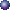# Visual Differential Equations

### 1. First Order Differential EquationsDirection Field appletNumerical Solutions: Euler's MethodNumerical Solutions: Step Size and AccuracyNumerical Solutions: Improved Euler's Method Example 1, Example 2

### 2. Boundary Value ProblemsDifferent Boundary Conditions in 1-D2-D Modes with Circular Boundary
mode m=0,n=1
mode m=0,n=2
mode m=1,n=1
mode m=2,n=3
mode m=5,n=4